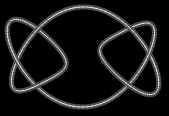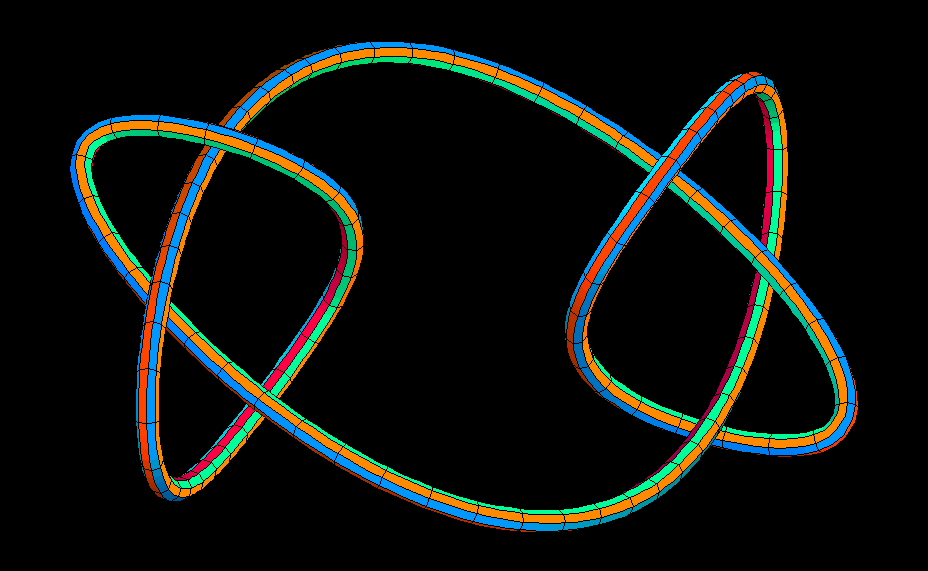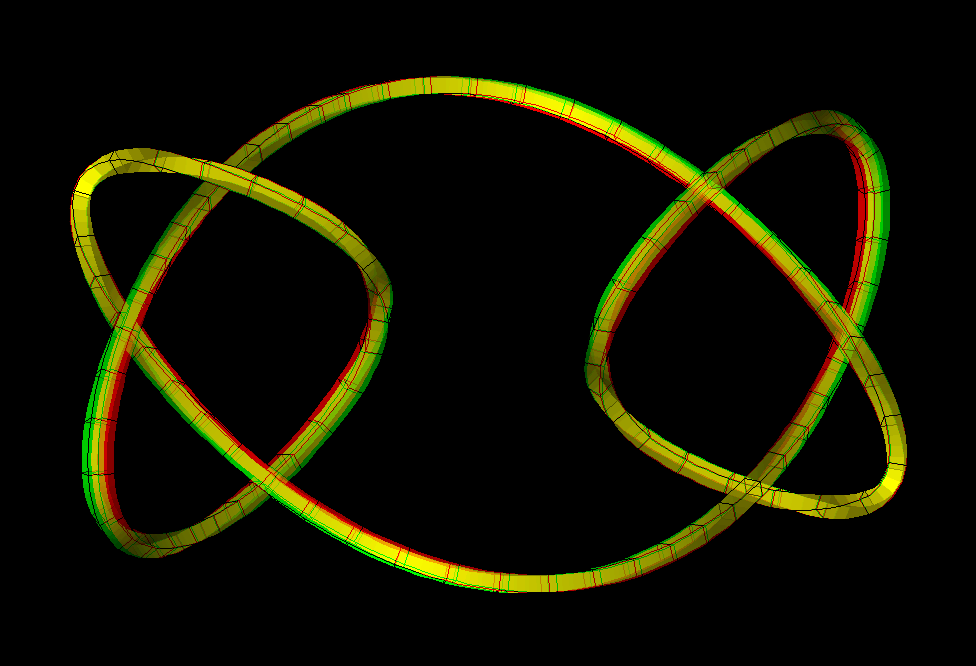# Square Knot

Back to interactive square_knotThe Square Knot is the so called knot sum of two mirror symmetric Trefoil Knots.Square Knot, rotating around a vertical axis. Note that the square knot is symmetric with respect to the origin. It has 180 degree rotation symmetry about the x-axis and the composition of these two symmetries is reflection in the y-z-plane. Compare with the Granny Knot.Square Knot, rotating view. (anaglyph)

Square_Knot.pdf